## Newton's Laws Applications Review

### Part A: Drawing Free-Body Diagrams

1. Construct free-body diagrams for the following physical situations.

 a. A ball is dropped from rest from the top of a building. Assume negligible air resistance. b. After being thrown, a football is moving upwards and rightwards towards the peak of its trajectory. Assume negligible air resistance. c. After reaching a terminal velocity, a falling skydiver then opens up the parachute. d. An air track glider is gliding to the right at constant velocity. e. A car is skidding to a stop while traveling to the right. f. A downward moving elevator (held by a cable) slows down. g. A 25.0-N force is applied at a 30-degree angle to a crate in order to accelerate it rightward across a rough, horizontal surface. h. A picture hangs symmetrically by two wires oriented at angles to the vertical. i. A large crate slowly accelerated down a steep and rough inclined plane.

### Part B: Conceptual Notions of Force and Motion

Express your understanding of concepts of force, acceleration, and motion by answering the following questions.

2. Which of the following are always true of an object that is at equilibrium? Include all that apply.

1. All the forces acting upon the object are equal.
2. The object is at rest.
3. The object is moving and moving with a constant velocity.
4. The object has an acceleration of zero.
5. There is no change in the object's velocity.
6. The sum of all the forces is 0 N.
7. All the forces acting upon an object are balanced.

3. Which of the following are never true of an object that is at equilibrium? Include all that apply.

1. The object is accelerating.
2. The object is at rest.
3. The object is moving in a circle at constant speed.
4. All the forces acting upon the object are equal.
5. The object is in free-fall.
6. The object is falling and has reached its terminal velocity.
7. There is a net force acting upon the object.
8. The object is moving and moving with a constant velocity.
9. The object is at rest.

4. Big Bubba has a mass of 100 kg on the earth. What is Big Bubba's mass on the moon where the force of gravity is approximately 1/6-th that of Earth's? ________ Explain or show your work.

5. Little Billie weight 360 N on Earth. What is Little Billie's mass on the moon where the force of gravity is approximately 1/6-th that of Earth's? ________ Explain or show your work.

6. TRUE or FALSE:

An object which is moving rightward has a rightward force acting upon it.

7. How much net force is required to keep a 5-kg object moving rightward with a constant velocity of 2 m/s? ________ Explain or show your work.

8. TRUE or FALSE:

For an object resting upon a non-accelerating surface, the normal force is equal to the force of gravity.

9. An object rests upon an inclined plane. If the angle of incline is increased, then the normal force would _______ .

 a. increase b. decrease c. remain the same

10. Three pictures of equal weight (20 N) are hung by wires in three different orientations. In which orientation are the wires least likely to break? _______ Explain why.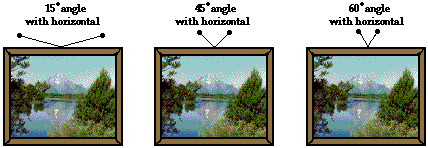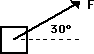11. A 50-N force is applied at an angle of 30 degrees north of east. This would be the same as applying two forces at

1. 43 N, east and 7 N, north
2. 35 N, east and 15 N, north
3. 25 N, east and 25 N, north
4. 43 N, east and 25 N, north

12. Which one(s) of the following force diagrams depict an object moving to the right with constant speed? Write all possible answers (if any) in the blank: ________________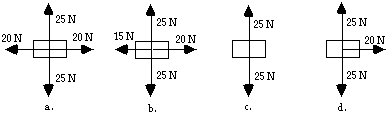### Part C: Force-Mass-Acceleration Relationships

Use the approximation that g=~10 m/s2 to fill in the blanks in the following diagrams.

 13.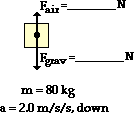14.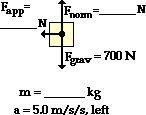15.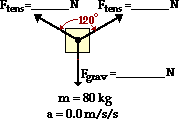16.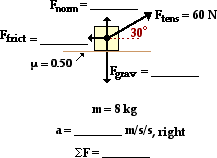17.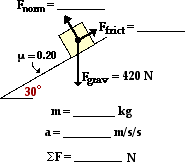18.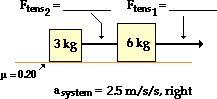19.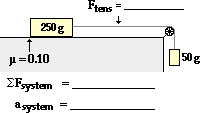20.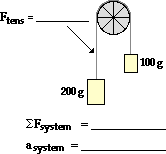21.### Part D: Problem-Solving

22. A 945-kg car traveling rightward at 22.6 m/s slams on the brakes and skids to a stop (with locked wheels). If the coefficient of friction between tires and road is 0.972, determine the distance required to stop. PSYW

23. A student pulls a 2-kg backpack across the ice (assume frictionless) by pulling at a 30.0 degrees angle to the horizontal. The velocity-time graph for the motion is shown. Perform a careful analysis of the situation and determine the applied force. PSYW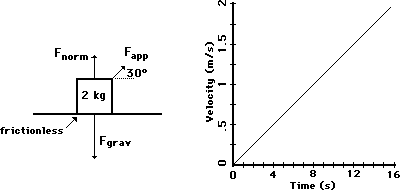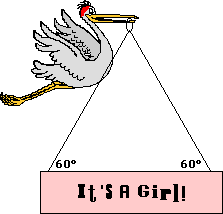24. After its most recent delivery, the infamous stork announces the good news. If the sign has a mass of 10.0 kg, then what is the tensional force in each cable? PSYW

25. Splash Mountain at Disney World in Orlando, Florida is one of the steepest water plume rides in the United States. Occupants of the boat fall from a height of 100 feet (3.2 ft = 1 m) down a wet ramp which makes a 45 degree angle with the horizontal. Consider the mass of the boat and its occupants to be 1500 kg. The coefficient of friction between the boat and the ramp is 0.10. Determine the frictional force, the acceleration, the distance traveled along the incline, and the final velocity of the boat at the bottom of the incline. PSYW

26. At last year's Homecoming Pep Rally, Trudy U. Skool (attempting to generate a little excitement) slid down a 42.0 degree incline from the sports dome to the courtyard below. The coefficient of friction between Trudy's jeans and the incline was 0.650. Determine Trudy's acceleration along the incline. Begin with a free-body diagram. PSYW

27. Baldwin Young is conducting his famous toupee experiments. He tips his head at a given angle and determines the coefficient of static and kinetic friction between a toupee (which is probably his own) and his scalp. At an angle of just barely 17.5 degrees, the toupee begins to accelerate from rest. Then Baldwin lowers the angle to 13.8 degrees to observe that the toupee moves with a constant speed. Use this information to determine the coefficients of both static and kinetic friction. Begin with a free-body diagram. PSYW

28. Consider the two-body system at the right. There is a 0.250-kg object accelerating across a rough surface. The sliding object is attached by a string to a 0.100 kg object which is suspended over a pulley. The coefficient of kinetic friction is 0.183. Calculate the acceleration of the block and the tension in the string. PSYW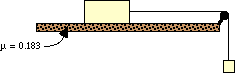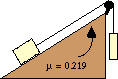29. Consider the two-body system at the right. A 22.7-N block is placed upon an inclined plane which is inclined at a 17.2 degree angle. The block is attached by a string to a 34.5-N block which is suspended over a pulley. The coefficient of friction is 0.219. Determine the acceleration of the block and the tension in the string. PSYW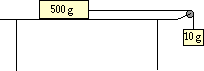30. A 10.0-gram mass is tied to a string.  The string is attached to a 500.0-gram mass and stretched over a pulley, leaving the 10.0-gram mass suspended above the floor.  Determine the time it will take the 10.0-gram mass to fall a distance of 1.50 meters if starting from rest. (No friction)

#### Navigate to:

Review Session Home - Topic Listing

Forces in 2 Dimensions - Home || Printable Version || Questions with Links

Answers to Questions:  All || #1-12 || #13-21 || #22-30

## You Might Also Like ...

Users of The Review Session are often looking for learning resources that provide them with practice and review opportunities that include built-in feedback and instruction. If that is what you're looking for, then you might also like the following:

The Calculator Pad includes physics word problems organized by topic. Each problem is accompanied by a pop-up answer and an audio file that explains the details of how to approach and solve the problem. It's a perfect resource for those wishing to improve their problem-solving skills.

Visit: The Calculator Pad Home | Calculator Pad - Forces in Two Dimensions

2. Minds On Physics the App Series

Minds On Physics the App ("MOP the App") is a series of interactive questioning modules for the student that is serious about improving their conceptual understanding of physics. Each module of the series covers a different topic and is further broken down into sub-topics. A "MOP experience" will provide a learner with challenging questions, feedback, and question-specific help in the context of a game-like environment. It is available for phones, tablets, Chromebooks, and Macintosh computers. It's a perfect resource for those wishing to refine their conceptual reasoning abilities. Part 2 of the series includes Forces in Two Dimensions.

Visit: MOP the App Home || MOP the App - Part 2